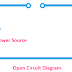According to the presence of complete or incomplete path for the flow of electric current, electrical or electronic circuit divided into two types - 1. Open Circuit 2. Closed Circuit. In the basics of electrical or electronics engineering, so it is must know the clear concept of open circuits and closed circuits. So, in this article, we are going to know the basics concept of open circuits and closed circuits, their characteristics, circuit diagram, difference, etc.

## What is Open Circuit?

An electrical or electronic circuit where no complete path for the electric current to flow from the power source to load or return from the load to the source is called an open circuit. An open circuit has one or multiple broken paths for the flow of electric current. Even, when an electrical or electronic circuit has at least one broken path, it will be called an open circuit.

The definition of an open circuit is also be expressed in different words such as, an open circuit is the electrical or electronic circuit where electric current or electric energy does not flow or transferred.

## Diagram of Open Circuit

Here, you can see an open circuit diagram.

You can see, here the power source is a battery and the load is the lamp. You can see there is a broken path. So current cannot flow from the battery to the lamp. That's why it is considered as an open circuit.

## Open Circuit Characteristics

The main characteristics of an open circuit are,

1. There is no flow of current from the power source to load.

2. Electrical energy does not transfer from source to load.

3. Open circuits do not any useful work.

4. There is no electrical power loss happens as no current flow in the circuit.

5. There is no voltage drop in the open circuit.

## Open Circuit Example, Causes, Applications

A circuit with a switch-off condition can be called an example of open circuit.

There are multiple causes that may be available for the open circuit such as,
1. Switched Off Condition.
2. The fuse connected to the circuit may be blown.
3. Faulty Wiring Connection.
4. Missing Circuit Component.

There are no practical applications of open circuit as it do not have any useful work. Only experiment purpose or measurement purpose open circuit may use in laboratories.

## What is Closed Circuit?

The electrical or electronic circuit where a complete path for the flow of electric current is available is called closed-circuit or we can say the circuit without having any broken path is called a closed circuit.
In a closed circuit, current can flow from the source to load and also be returned from the load to the source.

The definition of closed-circuit also be expressed as different words such as the circuit where electrical energy can transfer easily from the source to load is called Closed Circuit. Actually, all the electrical or electronic circuits do the useful works or subjected to proper operation is marked as a closed circuit.

## Closed Circuit Diagram

Here, you can see the diagram of closed circuit.

Here, you can see the power source is a battery and the load is a lamp. Both the battery and lamp are connected properly by a closed path. So the electric current can flow from the battery to the lamp. There is no broken path, so the lamp can glow. Also, see, the electrical energy can easily be transferred from the battery to the lamp.

## Closed Circuit Characteristics

Some important characteristics of Closed Circuit are,
1. Electric Current can easily flow from the power source to load.
2. Electrical Energy can easily be transferred from the source to the load.
3. There is electrical power loss(copper loss) that happens due to the internal resistance of the conductor and circuit component to the flow of electric current.
4. In a closed circuit, always voltage drop occurs due to the flow of current and internal resistance of the circuit or component.

## Closed Circuit Example, Causes, Applications

All the electrical and electronic circuits subjected to operation are examples of closed circuit.

As all the components and power sources are connected in a closed-loop, it is called a closed circuit. So, connecting all the components and power sources by closing all ends causes to the creation of a closed circuit.

All the lighting circuits, motor circuits, internal circuits of the electrical and electronic appliances are the applications of closed circuit.

## Difference Between Open Circuit and Closed Circuit

1. The main difference between an open circuit and a closed circuit is, there is no broken path in a closed circuit whereas the open circuit has at least one broken path.

2. There is no flow of current in an open circuit whereas, in a closed circuit, current flows from the source to load in a circular way.

3. Electrical energy transfer is not possible in an open circuit but in a closed energy transfer possible from source to load.

4. There is no voltage drop in an open circuit but a voltage drop occurs in a closed circuit.

5. There is no power loss that happens in an open circuit but power loss happens in a closed circuit.

Thank you for visiting the website. keep visiting for more updates.

Open Circuit VS Closed Circuit Diagram, CharacteristicsReviewed by Author on 7/28/2021 Rating: 5